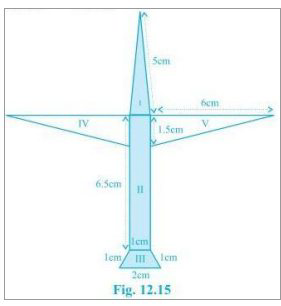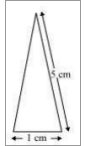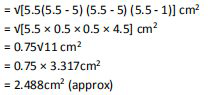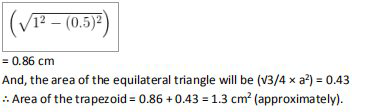# NCERT Solutions Class 9 Mathematics Solutions for Heron’s Formula - Exercise 12.2 in Chapter 12 - Heron’s Formula

Radha made a picture of an airplane with colored paper as shown in Fig 12.15. Find the

the total area of the paper used.For the triangle I section:It is an isosceles triangle and the sides are 5 cm, 1 cm and 5 cm

Perimeter = 5 + 5 + 1 = 11 cm

So, semi perimeter = 11/2 cm = 5.5 cm

Using Heron's formula,

Area = √[s (s-a) (s-b) (s-c)]

,This quadrilateral is a rectangl with length and breadth as 6.5 cm and 1 cm respectively.

\therefore \text { Area }=6.5 \times 1 \mathrm{cm}^{2}=6.5 \mathrm{cm}^{2}

It is a trapezoid with 2 sides as 1 cm each and the third side as 2 cm.

Area of the trapezoid = Area of the parallelogram + Area of the equilateral triangle

The perpendicular height of the parallelogram will beFor triangle IV and V:

These triangles are 2 congruent right-angled triangles having the base as 6 cm and height

1.5 cm

\begin{aligned} &\text { Area triangles } \mathrm{IV} \text { and } \mathrm{V}=2 \times(7 / 2 \times 6 \times 1.5) \mathrm{cm}^{2}=9 \mathrm{cm}^{2}\\ &\text { So, the total area of the paper used }=(2.488+6.5+1.3+9) \mathrm{cm}^{2}=19.3 \mathrm{cm}^{2} \end{aligned}

Related Questions

Lido

Courses

Teachers

Book a Demo with us

Syllabus

Maths
CBSE
Maths
ICSE
Science
CBSE

Science
ICSE
English
CBSE
English
ICSE
Coding

Terms & Policies

Selina Question Bank

Maths
Physics
Biology

Allied Question Bank

Chemistry
Connect with us on social media!